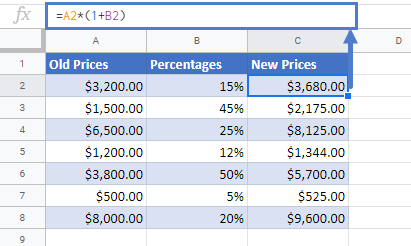# Calculate Percentage Increase in Excel & Google Sheets

This tutorial will demonstrate how to calculate the percentage increase (or increase a number by a percentage) in Excel & Google Sheets.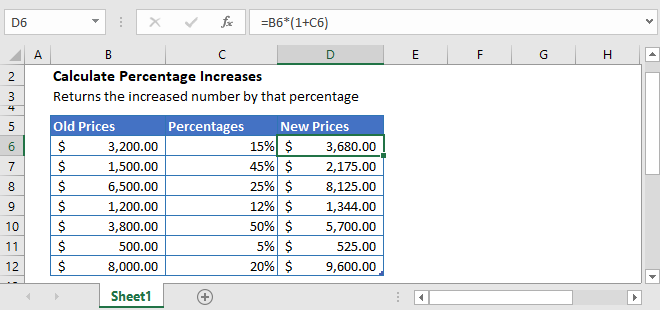## Percentage Increase Formula

To increase a number by a specified percentage, simply multiply that number by 1 plus the percentage increase.

For example, to increase a product’s price by 15% (which was \$250), use this formula:

=\$250*(1+15%)

=\$250*(115%)

=\$287.5

After calculation, the new price is \$287.50.

## General Formula

We can generalize the formula to calculate the percentage increase as:

``=number*(1+percentage_increase)``

## Increase Product Prices by Specific Percentages

Let’s that you want to increase your product prices by certain percentages. The old prices of the products and their respective increase percentages are mentioned in the table below.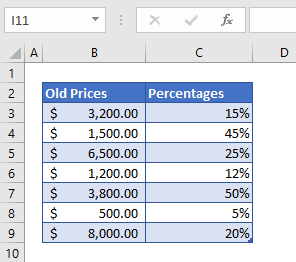We have the old prices of the products and now to calculate the new prices we are simply going to apply the percentage increase formula in cell D3.

``=B3*(1+C3)``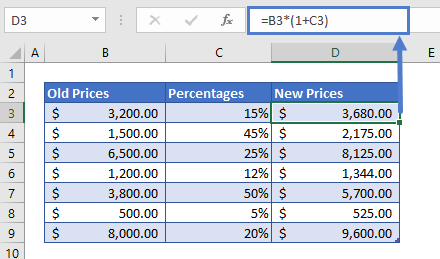To help you better understand the formula, we have divided the above formula into three simple steps:

### Step 1

In the above table, we have the old prices of products, and their respective percentage increases. In the first step, we are going to add 1 to the percentages.

``=(1+C3)``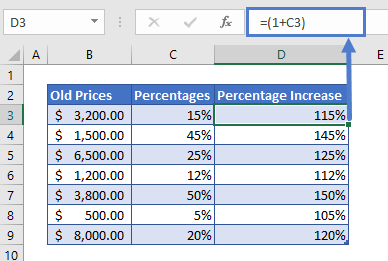### Step 2

Now that we have the correct percentages, we are going to multiply these percentages with their respective old prices. This will give us our new prices with the percentage increase.

``=B3*D3``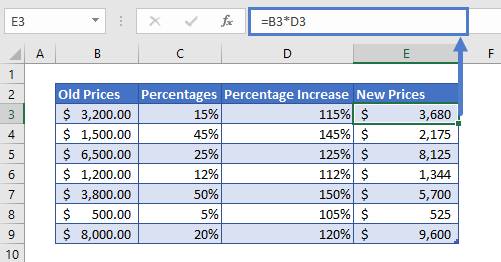Let’s that you want to increase all your product prices by 5%. For this, you’ll have to apply the percentage increase formula.

As all the product prices need to be increased by 5%. So we going to use absolute reference for the percentage increase cell F2. To fix this cell (F2), we have to select the cell F2 in the formula bar and press the F4 key to make it look like \$F\$2.

``=B3*(1+\$F\$2)``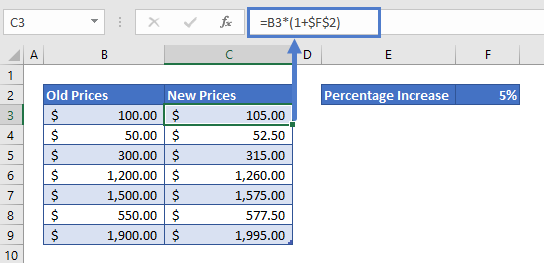### Easy Excel Automation